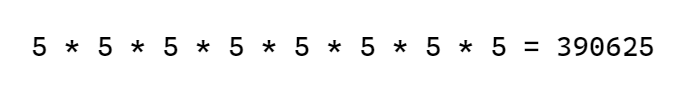< 返回

二进制取幂（快速幂）

2021年8月14日

# 循环取幂// 5^8
val x = 5
val n = 8
var r = x

for (i in 1 until n) {
r *= x
}

println(r) // 390625

# 更省力的手算# 快速幂的代码实现fun pow(x:Long, n:Long) : Long {
if (n == 0L) return 1;
val res = pow(x, n / 2)
return res * res * if (n % 2 == 1L) x else 1
}

fun binaryPow(_x:Long, _n:Long) : Long {
var x = _x
var n = _n
var res = 1L
while (n > 0) {
// n & 1
if (n.and(1) == 1L) res *= x
x *= x
// n >> 1
n = n ushr 1
}
return res
}